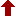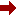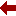#### SLIM21

 Sort Order Chronological Author Title ItemType,Author ItemType,Title Display Format Tabular Publication Bibliography Items / Page 5 10 25

Click the serial number on the left to view the details of the item.
#  AuthorTitleAccn#YearItem Type Claims
11 Kantorovich, Lev Mathematics for Natural Scientists II I10062 2016 eBook
12 Belhaq, Mohamed Recent Trends in Applied Nonlinear Mechanics and Physics I09989 2018 eBook
13 Chui, Charles K Kalman Filtering I09935 2017 eBook
14 Sagaut, Pierre Homogeneous Turbulence Dynamics I09923 2018 eBook
15 ??irca, Simon Computational Methods in Physics I09821 2018 eBook
16 Kundu, Sumit Proceedings of the 2nd International Conference on Communication, Devices and Computing I09710 2020 eBook
17 Landi, Giovanni Linear Algebra and Analytic Geometry for Physical Sciences I09687 2018 eBook
18 Chong, Christopher Coherent Structures in Granular Crystals I09682 2018 eBook
19 Scheck, Florian Mechanics I09673 2018 eBook
20 Maximon, Leonard C Differential and Difference Equations I09655 2016 eBook
 (page:2 / 32) [#317] First Page Previous Page Next Page Last Page

11.TitleMathematics for Natural Scientists II : Advanced Methods
Author(s)Kantorovich, Lev
PublicationCham, 1. Imprint: Springer 2. Springer International Publishing, 2016.
DescriptionXVII, 675 p. 107 illus., 104 illus. in color : online resource
Abstract NoteThis book covers the advanced mathematical techniques useful for physics and engineering students, presented in a form accessible to physics students, avoiding precise mathematical jargon and laborious proofs. Instead, all proofs are given in a simplified form that is clear and convincing for a physicist. Examples, where appropriate, are given from physics contexts. Both solved and unsolved problems are provided in each chapter. Mathematics for Natural Scientists II: Advanced Methods is the second of two volumes. It follows the first volume on Fundamentals and Basics
ISBN,Price9783319278612
Keyword(s)1. APPLIED MATHEMATICS 2. Chemometrics 3. EBOOK 4. EBOOK - SPRINGER 5. ENGINEERING MATHEMATICS 6. Math. Applications in Chemistry 7. Mathematical and Computational Engineering 8. Mathematical Applications in the Physical Sciences 9. Mathematical Methods in Physics 10. MATHEMATICAL PHYSICS 11. Numerical and Computational Physics, Simulation 12. PHYSICS
Item TypeeBook
Circulation Data
 Accession# Call# Status Issued To Return Due On Physical Location I10062 On Shelf

12.TitleRecent Trends in Applied Nonlinear Mechanics and Physics : Selected Papers from CSNDD 2016
Author(s)Belhaq, Mohamed
PublicationCham, 1. Imprint: Springer 2. Springer International Publishing, 2018.
DescriptionXI, 278 p. 143 illus., 90 illus. in color : online resource
Abstract NoteThis book presents contributions on the most active lines of recent advanced research in the field of nonlinear mechanics and physics selected from the 4th International Conference on Structural Nonlinear Dynamics and Diagnosis. It includes fifteen chapters by outstanding scientists, covering various aspects of applications, including road tanker dynamics and stability, simulation of abrasive wear, energy harvesting, modeling and analysis of flexoelectric nanoactuator, periodic Fermi???Pasta???Ulam problems, nonlinear stability in Hamiltonian systems, nonlinear dynamics of rotating composites, nonlinear vibrations of a shallow arch, extreme pulse dynamics in mode-locked lasers, localized structures in a photonic crystal fiber resonator, nonlinear stochastic dynamics, linearization of nonlinear resonances, treatment of a linear delay differential equation, and fractional nonlinear damping. It appeals to a wide range of experts in the field of structural nonlinear dynamics and offers researchers and engineers an introduction to the challenges posed by nonlinearities in the development of these topics
ISBN,Price9783319639376
Keyword(s)1. Applications of Nonlinear Dynamics and Chaos Theory 2. APPLIED MATHEMATICS 3. Computational Science and Engineering 4. Computer mathematics 5. DYNAMICAL SYSTEMS 6. DYNAMICS 7. EBOOK 8. EBOOK - SPRINGER 9. ENGINEERING MATHEMATICS 10. Mathematical and Computational Engineering 11. MECHANICS 12. Mechanics, Applied 13. Solid Mechanics 14. STATISTICAL PHYSICS 15. VIBRATION 16. Vibration, Dynamical Systems, Control
Item TypeeBook
Circulation Data
 Accession# Call# Status Issued To Return Due On Physical Location I09989 On Shelf

13.TitleKalman Filtering : with Real-Time Applications
Author(s)Chui, Charles K;Chen, Guanrong
PublicationCham, 1. Imprint: Springer 2. Springer International Publishing, 2017.
DescriptionXVIII, 247 p. 34 illus : online resource
Abstract NoteThis new edition presents a thorough discussion of the mathematical theory and computational schemes of Kalman filtering. The filtering algorithms are derived via different approaches, including a direct method consisting of a series of elementary steps, and an indirect method based on innovation projection. Other topics include Kalman filtering for systems with correlated noise or colored noise, limiting Kalman filtering for time-invariant systems, extended Kalman filtering for nonlinear systems, interval Kalman filtering for uncertain systems, and wavelet Kalman filtering for multiresolution analysis of random signals. Most filtering algorithms are illustrated by using simplified radar tracking examples. The style of the book is informal, and the mathematics is elementary but rigorous. The text is self-contained, suitable for self-study, and accessible to all readers with a minimum knowledge of linear algebra, probability theory, and system engineering. Over 100 exercises and problems with solutions help deepen the knowledge. This new edition has a new chapter on filtering communication networks and data processing, together with new exercises and new real-time applications
ISBN,Price9783319476124
Keyword(s)1. APPLIED MATHEMATICS 2. ARTIFICIAL INTELLIGENCE 3. Communications Engineering, Networks 4. EBOOK 5. EBOOK - SPRINGER 6. ECONOMIC THEORY 7. Economic Theory/Quantitative Economics/Mathematical Methods 8. ELECTRICAL ENGINEERING 9. ENGINEERING MATHEMATICS 10. Mathematical and Computational Engineering 11. Mathematical Methods in Physics 12. Numerical and Computational Physics, Simulation 13. PHYSICS
Item TypeeBook
Circulation Data
 Accession# Call# Status Issued To Return Due On Physical Location I09935 On Shelf

14.TitleHomogeneous Turbulence Dynamics
Author(s)Sagaut, Pierre;Cambon, Claude
PublicationCham, 1. Imprint: Springer 2. Springer International Publishing, 2018.
DescriptionXXIX, 897 p. 267 illus., 75 illus. in color : online resource
Abstract NoteThis book provides state-of-the-art results and theories in homogeneous turbulence, including anisotropy and compressibility effects with extension to quantum turbulence, magneto-hydodynamic turbulence?? and turbulence in non-newtonian fluids. Each chapter is devoted to a given type of interaction (strain, rotation, shear, etc.), and presents and compares experimental data, numerical results, analysis of the Reynolds stress budget equations and advanced multipoint spectral theories. The role of both linear and non-linear mechanisms is emphasized. The link between the statistical properties and the dynamics of coherent structures is also addressed. Despite its restriction to homogeneous turbulence, the book is of interest to all people working in turbulence, since the basic physical mechanisms which are present in all turbulent flows are explained. The reader will find a unified presentation of the results and a clear presentation of existing controversies. Special attention is given to bridge the results obtained in different research communities. Mathematical tools and advanced physical models are detailed in dedicated chapters
ISBN,Price9783319731629
Keyword(s)1. APPLIED MATHEMATICS 2. EBOOK 3. EBOOK - SPRINGER 4. Engineering Fluid Dynamics 5. ENGINEERING MATHEMATICS 6. FLUID MECHANICS 7. Fluid- and Aerodynamics 8. FLUIDS 9. Mathematical and Computational Engineering 10. Mathematical Applications in the Physical Sciences 11. MATHEMATICAL PHYSICS 12. Theoretical, Mathematical and Computational Physics
Item TypeeBook
Circulation Data
 Accession# Call# Status Issued To Return Due On Physical Location I09923 On Shelf

15.TitleComputational Methods in Physics : Compendium for Students
Author(s)Sirca, Simon;Horvat, Martin
PublicationCham, 1. Imprint: Springer 2. Springer International Publishing, 2018.
DescriptionXXIV, 880 p. 268 illus., 20 illus. in color : online resource
Abstract NoteThis book is intended to help advanced undergraduate, graduate, and postdoctoral students in their daily work by odering them a compendium of numerical methods. The choice of methods pays signi???cant attention to error estimates, stability and convergence issues, as well as optimization of program execution speeds. Numerous examples are given throughout the chapters, followed by comprehensive end-of-chapter problems with a more pronounced physics background, while less stress is given to the explanation of individual algorithms. The readers are encouraged to develop a certain amount of skepticism and scrutiny instead of blindly following readily available commercial tools. The second edition has been enriched by a chapter on inverse problems dealing with the solution of integral equations, inverse Sturm-Liouville problems, as well as retrospective and recovery problems for partial di???erential equations. The revised text now includes an introduction to sparse matrix methods, the solution of matrix equations, and pseudospectra of matrices; it discusses the sparse Fourier, non-uniform Fourier and discrete wavelet transformations, the basics of non-linear regression and the Kolmogorov-Smirnov test; it demonstrates the key concepts in solving sti??? di???erential equations and the asymptotics of Sturm-Liouville eigenvalues and eigenfunctions. Among other updates, it also presents the techniques of state-space reconstruction, methods to calculate the matrix exponential, generate random permutations and compute stable derivatives
ISBN,Price9783319786193
Keyword(s)1. APPLIED MATHEMATICS 2. Chemistry, Physical and theoretical 3. Computational Science and Engineering 4. Computer mathematics 5. EBOOK 6. EBOOK - SPRINGER 7. ENGINEERING MATHEMATICS 8. Mathematical and Computational Engineering 9. Numerical and Computational Physics, Simulation 10. PHYSICS 11. Theoretical and Computational Chemistry
Item TypeeBook
Circulation Data
 Accession# Call# Status Issued To Return Due On Physical Location I09821 On Shelf

16.TitleProceedings of the 2nd International Conference on Communication, Devices and Computing : ICCDC 2019
Author(s)Kundu, Sumit;Acharya, U. Shripathi;De, Chanchal Kr;Mukherjee, Surajit
PublicationSingapore, 1. Imprint: Springer 2. Springer Singapore, 2020.
DescriptionXXI, 735 p. 481 illus., 300 illus. in color : online resource
Abstract NoteThis book gathers high-quality papers presented at the 2nd International Conference on Communication, Devices & Computing (ICCDC 2019), held at Haldia Institute of Technology from March 14???15, 2019. The papers are divided into three main areas: communication technologies, electronics circuits & devices and computing. Written by students and researchers from around the world, they accurately reflect the global status quo
ISBN,Price9789811508295
Keyword(s)1. APPLIED MATHEMATICS 2. CIRCUITS AND SYSTEMS 3. EBOOK 4. EBOOK - SPRINGER 5. ELECTRONIC CIRCUITS 6. Electronic Circuits and Devices 7. ENGINEERING MATHEMATICS 8. Mathematical and Computational Engineering
Item TypeeBook
Circulation Data
 Accession# Call# Status Issued To Return Due On Physical Location I09710 On Shelf

17.TitleLinear Algebra and Analytic Geometry for Physical Sciences
Author(s)Landi, Giovanni;Zampini, Alessandro
PublicationCham, 1. Imprint: Springer 2. Springer International Publishing, 2018.
DescriptionXII, 345 p : online resource
Abstract NoteA self-contained introduction to finite dimensional vector spaces, matrices, systems of linear equations, spectral analysis on euclidean and hermitian spaces, affine euclidean geometry, quadratic forms and conic sections. The mathematical formalism is motivated and introduced by problems from physics, notably mechanics (including celestial) and electro-magnetism, with more than two hundreds examples and solved exercises. Topics include: The group of orthogonal transformations on euclidean spaces, in particular rotations, with Euler angles and angular velocity. The rigid body with its inertia matrix. The unitary group. Lie algebras and exponential map. The Dirac???s bra-ket formalism. Spectral theory for self-adjoint endomorphisms on euclidean and hermitian spaces. The Minkowski spacetime from special relativity and the Maxwell equations. Conic sections with the use of eccentricity and Keplerian motions. An appendix collects basic algebraic notions like group, ring and field; and complex numbers and integers modulo a prime number. The book will be useful to students taking a physics or engineer degree for a basic education as well as for students who wish to be competent in the subject and who may want to pursue a post-graduate qualification
ISBN,Price9783319783611
Keyword(s)1. ALGEBRA 2. APPLIED MATHEMATICS 3. Computer science???Mathematics 4. EBOOK 5. EBOOK - SPRINGER 6. ENGINEERING MATHEMATICS 7. GEOMETRY 8. Linear and Multilinear Algebras, Matrix Theory 9. Math Applications in Computer Science 10. Mathematical and Computational Engineering 11. Mathematical Applications in the Physical Sciences 12. Mathematical Methods in Physics 13. MATHEMATICAL PHYSICS 14. MATRIX THEORY 15. PHYSICS
Item TypeeBook
Circulation Data
 Accession# Call# Status Issued To Return Due On Physical Location I09687 On Shelf

18.TitleCoherent Structures in Granular Crystals : From Experiment and Modelling to Computation and Mathematical Analysis
Author(s)Chong, Christopher;Kevrekidis, Panayotis G
PublicationCham, 1. Imprint: Springer 2. Springer International Publishing, 2018.
DescriptionXII, 94 p. 35 illus., 29 illus. in color : online resource
Abstract NoteThis book summarizes a number of fundamental developments at the interface of granular crystals and the mathematical and computational analysis of some of their key localized nonlinear wave solutions. The subject presents a blend of the appeal of granular crystals as a prototypical engineering tested for a variety of diverse applications, the novelty in the nonlinear physics of its coherent structures, and the tractability of a series of mathematical and computational techniques to analyse them. While the focus is on principal one-dimensional solutions such as shock waves, traveling waves, and discrete breathers, numerous extensions of the discussed patterns, e.g., in two dimensions, chains with defects, heterogeneous settings, and other recent developments are discussed. The book appeals to researchers in the field, as well as for graduate and advanced undergraduate students. It will be of interest to mathematicians, physicists and engineers alike
ISBN,Price9783319778846
Keyword(s)1. Amorphous substances 2. APPLIED MATHEMATICS 3. Complex fluids 4. EBOOK 5. EBOOK - SPRINGER 6. ENGINEERING MATHEMATICS 7. Mathematical and Computational Engineering 8. Mathematical Applications in the Physical Sciences 9. Mathematical Methods in Physics 10. MATHEMATICAL PHYSICS 11. PHYSICS 12. Soft and Granular Matter, Complex Fluids and Microfluidics 13. Structural Materials
Item TypeeBook
Circulation Data
 Accession# Call# Status Issued To Return Due On Physical Location I09682 On Shelf

19.TitleMechanics : From Newton's Laws to Deterministic Chaos
Author(s)Scheck, Florian
PublicationBerlin, Heidelberg, 1. Imprint: Springer 2. Springer Berlin Heidelberg, 2018.
DescriptionXVII, 591 p. 175 illus : online resource
Abstract NoteThis book covers all topics in mechanics from elementary Newtonian mechanics, the principles of canonical mechanics and rigid body mechanics to relativistic mechanics and nonlinear dynamics. It was among the first textbooks to include dynamical systems and deterministic chaos in due detail. As compared to the previous editions the present 6th edition is updated and revised with more explanations, additional examples and problems with solutions, together with new??sections on applications in science. ?? Symmetries and invariance principles, the basic geometric aspects of mechanics as well as elements of continuum mechanics also play an important role. The book will enable the reader to develop general principles from which equations of motion follow, to understand the importance of canonical mechanics and of symmetries as a basis for quantum mechanics, and to get practice in using general theoretical concepts and tools that are essential for all branches of physics. ?? The book contains more than 150 problems with complete solutions, as well as some practical examples which make moderate use of personal computers. This will be appreciated in particular by students using this textbook to accompany lectures on mechanics. The book ends with some historical notes on scientists who made important contributions to the development of mechanics
ISBN,Price9783662554906
Keyword(s)1. Applications of Mathematics 2. APPLIED MATHEMATICS 3. CLASSICAL MECHANICS 4. Dynamical Systems and Ergodic Theory 5. DYNAMICS 6. EBOOK 7. EBOOK - SPRINGER 8. ENGINEERING MATHEMATICS 9. ERGODIC THEORY 10. MECHANICS 11. Mechanics, Applied 12. Theoretical and Applied Mechanics
Item TypeeBook
Circulation Data
 Accession# Call# Status Issued To Return Due On Physical Location I09673 On Shelf

20.TitleDifferential and Difference Equations : A Comparison of Methods of Solution
Author(s)Maximon, Leonard C
PublicationCham, 1. Imprint: Springer 2. Springer International Publishing, 2016.
DescriptionXV, 162 p : online resource
Abstract NoteThis book, intended for researchers and graduate students in physics, applied mathematics and engineering, presents a detailed comparison of the important methods of solution for linear differential and difference equations - variation of constants, reduction of order, Laplace transforms and generating functions - bringing out the similarities as well as the significant differences in the respective analyses. Equations of arbitrary order are studied, followed by a detailed analysis for equations of first and second order. Equations with polynomial coefficients are considered and explicit solutions for equations with linear coefficients are given, showing significant differences in the functional form of solutions of differential equations from those of difference equations. An alternative method of solution involving transformation of both the dependent and independent variables is given for both differential and difference equations. A comprehensive, detailed treatment of Green???s functions and the associated initial and boundary conditions is presented for differential and difference equations of both arbitrary and second order. A dictionary of difference equations with polynomial coefficients provides a unique compilation of second order difference equations obeyed by the special functions of mathematical physics. Appendices augmenting the text include, in particular, a proof of Cramer???s rule, a detailed consideration of the role of the superposition principal in the Green???s function, and a derivation of the inverse of Laplace transforms and generating functions of particular use in the solution of second order linear differential and difference equations with linear coefficients
ISBN,Price9783319297361
Keyword(s)1. APPLIED MATHEMATICS 2. Difference and Functional Equations 3. DIFFERENCE EQUATIONS 4. DIFFERENTIAL EQUATIONS 5. EBOOK 6. EBOOK - SPRINGER 7. ENGINEERING MATHEMATICS 8. FUNCTIONAL EQUATIONS 9. Mathematical and Computational Engineering 10. Mathematical Applications in the Physical Sciences 11. Mathematical Methods in Physics 12. MATHEMATICAL PHYSICS 13. ORDINARY DIFFERENTIAL EQUATIONS 14. PHYSICS
Item TypeeBook